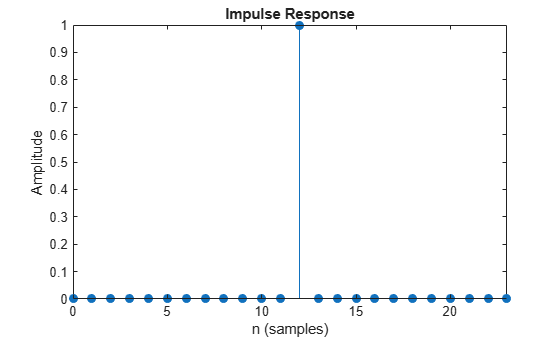# polyphase

Polyphase decomposition of multirate filter

## Syntax

``p = polyphase(sysobj)``
``p = polyphase(sysobj,'Arithmetic',arithType)``
``polyphase(sysobj)``

## Description

example

````p = polyphase(sysobj)` returns the polyphase matrix `p` of the multirate filter System object™ `sysobj`. Each row in the matrix corresponds to a polyphase branch. The number of columns in `p` corresponds to the number of filter taps per polyphase branch.```
````p = polyphase(sysobj,'Arithmetic',arithType)` returns the polyphase matrix `p` in the precision set by the `arithType`.```

example

````polyphase(sysobj)` launches the Filter Visualization Tool (fvtool) with all the polyphase subfilters to allow you to analyze each component subfilter individually.```

## Examples

collapse all

When you create a multirate filter that uses polyphase decomposition, polyphase lets you analyze the component filters individually by returning the components as rows in a matrix. First, create an interpolate-by-three filter.

`hs = dsp.FIRInterpolator`
```hs = dsp.FIRInterpolator with properties: InterpolationFactor: 3 NumeratorSource: 'Property' Numerator: [0 -1.2906e-04 -2.2804e-04 0 5.5461e-04 ... ] Show all properties ```

In this syntax, the matrix `p` contains all of the subfilters for `hm`, one filter per matrix row.

`p = polyphase(hs)`
```p = 3×24 0 0 0 0 0 0 0 0 0 0 0 0 1.0000 0 0 0 0 0 0 0 0 0 0 0 -0.0001 0.0006 -0.0015 0.0034 -0.0067 0.0121 -0.0205 0.0332 -0.0530 0.0861 -0.1540 0.4088 0.8247 -0.1976 0.1027 -0.0620 0.0388 -0.0241 0.0145 -0.0083 0.0043 -0.0020 0.0008 -0.0002 -0.0002 0.0008 -0.0020 0.0043 -0.0083 0.0145 -0.0241 0.0388 -0.0620 0.1027 -0.1976 0.8247 0.4088 -0.1540 0.0861 -0.0530 0.0332 -0.0205 0.0121 -0.0067 0.0034 -0.0015 0.0006 -0.0001 ```

Finally, using `polyphase` without an output argument opens the Filter Visualization Tool, ready for you to use the analysis capabilities of the tool to investigate the interpolator `hm`.

`polyphase(hs)`The fvtool shows the coefficients of the subfilters. To see the magnitude response of the subfilters, click on the `Magnitude Response` button on the fvtool toolstrip.

## Input Arguments

collapse all

Input filter, specified as as one of the following filter System objects:

Specify the arithmetic used in computing the polyphase matrix. When you specify `'double'` or `'single'`, the function performs double- or single-precision analysis. When you specify `'fixed'` , the arithmetic changes depending on the setting of the `CoefficientDataType` property and whether the System object is locked or unlocked.

Details for Fixed-Point Arithmetic

System Object StateCoefficient Data TypeRule
Unlocked`'Same as input'`The function assumes that the coefficient data type is signed, 16 bit, and autoscaled. The function performs fixed-point analysis based on this assumption.
Unlocked`'Custom'`The function performs fixed-point analysis based on the setting of the `CustomCoefficientsDataType` property.
Locked`'Same as input'`When the input data type is `'double'` or `'fixed'`, the function assumes that the coefficient data type is signed, 16-bit, and autoscaled. The function performs fixed-point analysis based on this assumption.
Locked`'Custom'`The function performs fixed-point analysis based on the setting of the `CustomCoefficientsDataType` property.

When you do not specify the arithmetic for non-CIC structures, the function uses double-precision arithmetic if the filter System object is in an unlocked state. If the System object is locked, the function performs analysis based on the locked input data type. CIC structures only support fixed-point arithmetic.

## Output Arguments

collapse all

Polyphase matrix `p` of the multirate filter. Each row in the matrix corresponds to a polyphase branch. The first row of matrix `p` represents the first polyphase branch, the second row the second polyphase branch, and so on to the last polyphase branch. The number of columns in `p` corresponds to the number of filter taps per polyphase branch.

## Version History

Introduced in R2011a